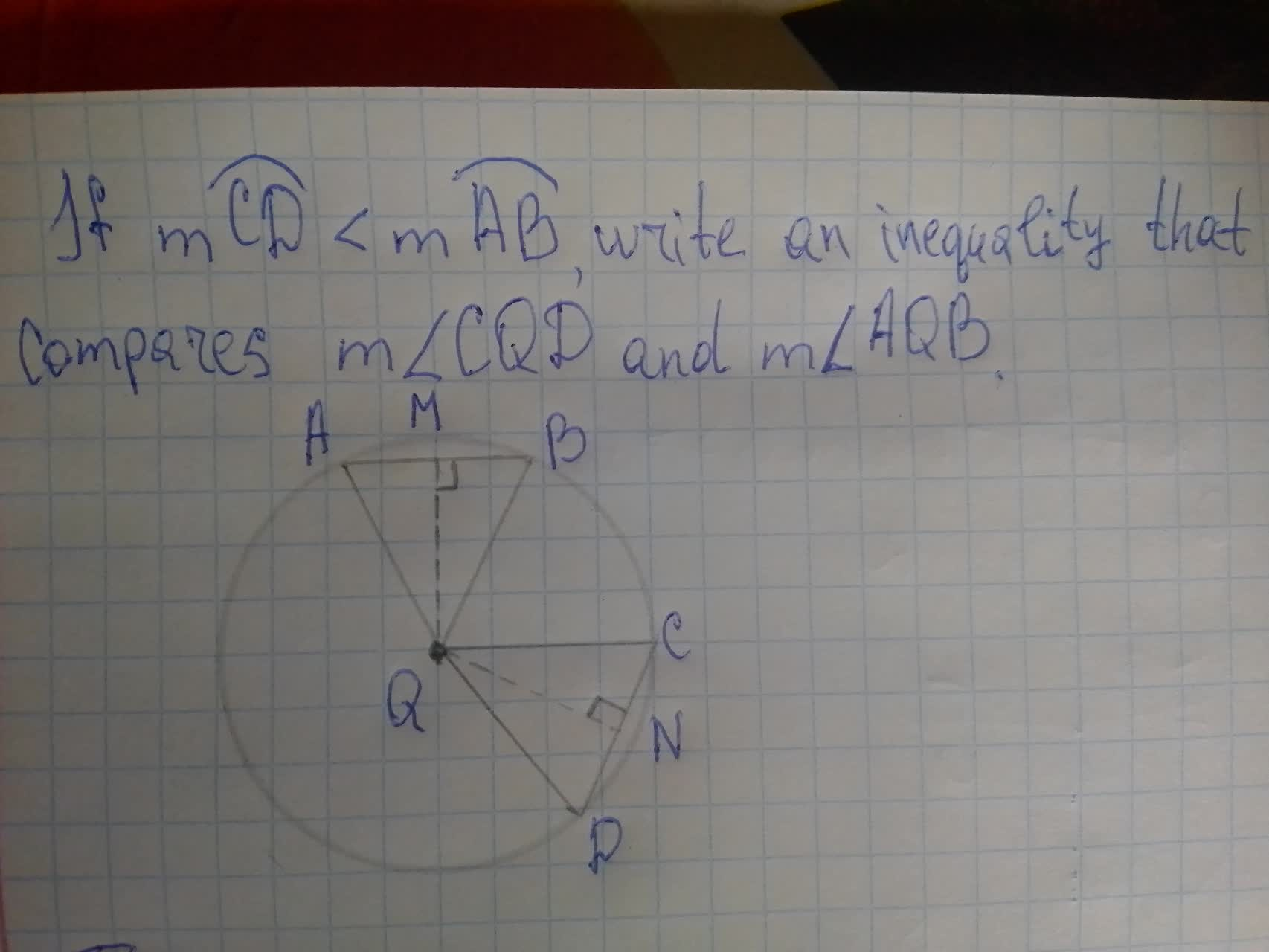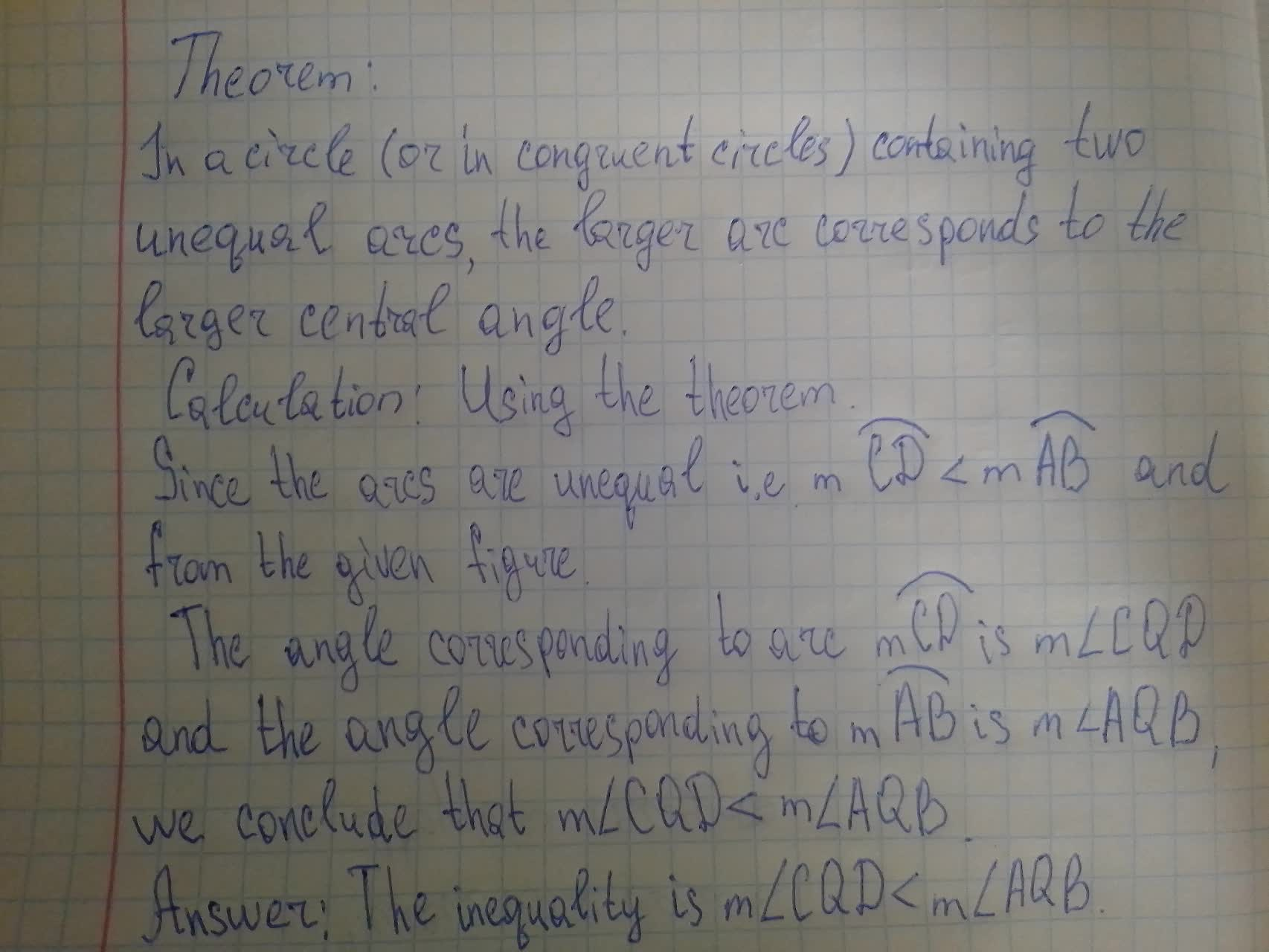Question# To write:An inequality that compares m\angle CQD and m\angle AQB

Circles
ANSWEREDTo write:
An inequality that compares m$$\displaystyle\angle{C}{Q}{D}$$ and m$$\displaystyle\angle{A}{Q}{B}$$